# What is conditional probability?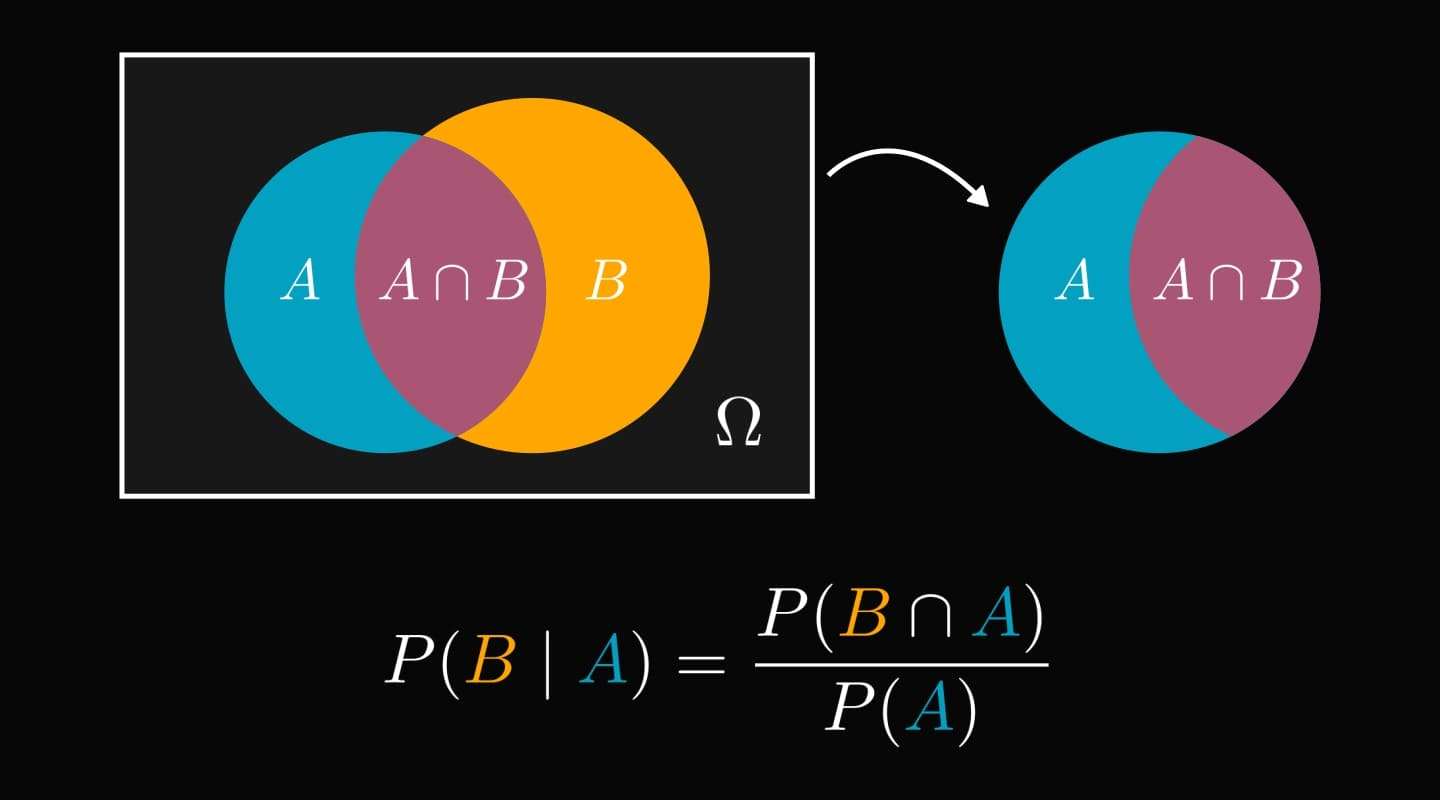Conditional probability is the single most important concept in statistics.

Why? Because without accounting for prior information, predictive models are useless.

Here is what conditional probability represents, and why it is essential.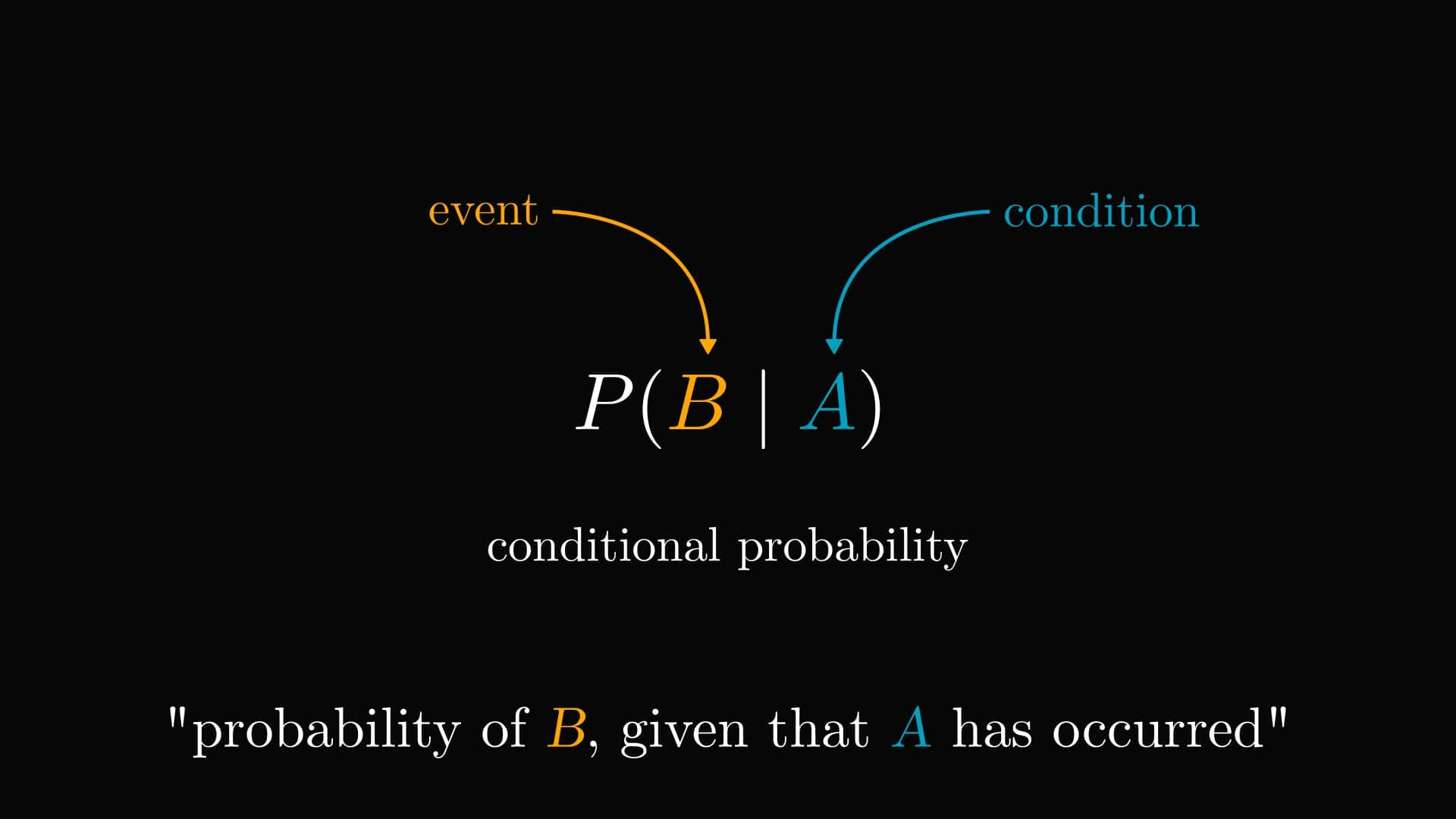Conditional probability allows us to update our models given new observations. By definition, $P(B \mid A)$ describes the probability of an event $B$, when $A$ has occurred.

Here is an example. Suppose that among $100$ people, $30$ have COVID.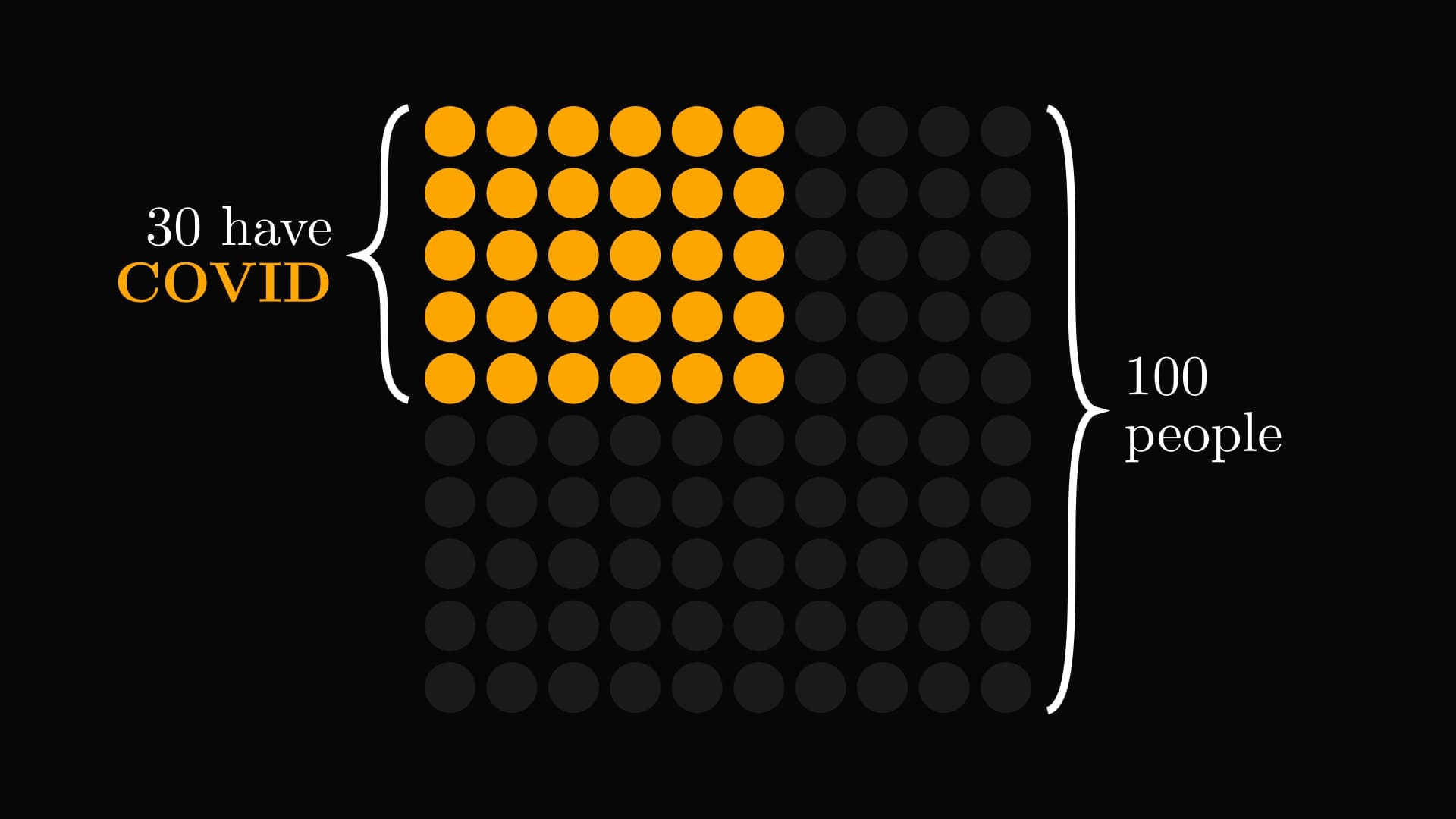Based only on this information, if we inspect a random person, our best guess is a $30\%$ chance of them having COVID.

This is not good enough. We can take a look at more information.

What about people exhibiting a symptom, like a fever?

It turns out that among the $100$ people, $40$ have a fever.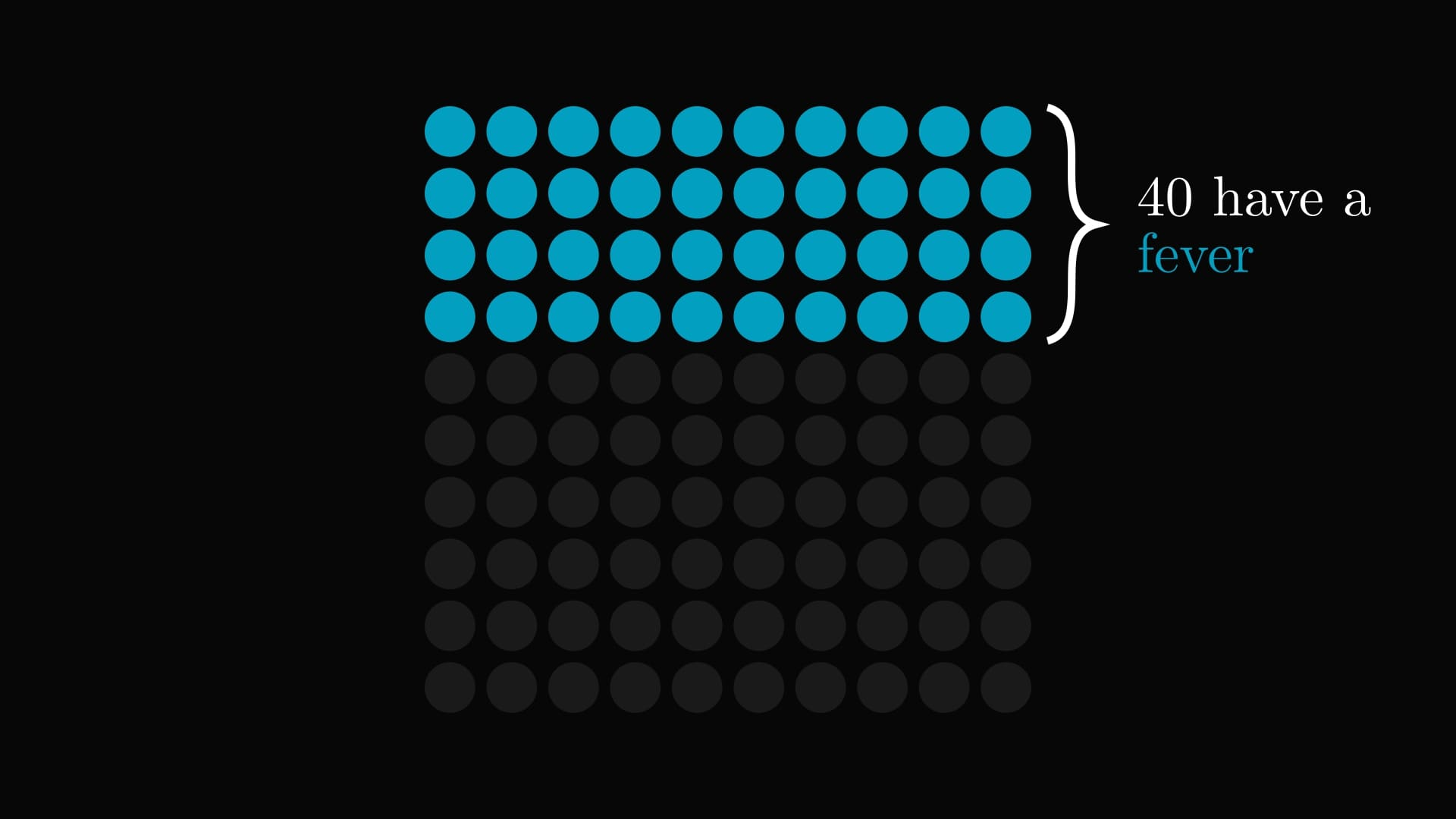Let's say that a random person with a high fever walks in. How does our probabilistic model change?

We can leverage the prior information (fever) to get a more precise diagnosis than the random $30\%$.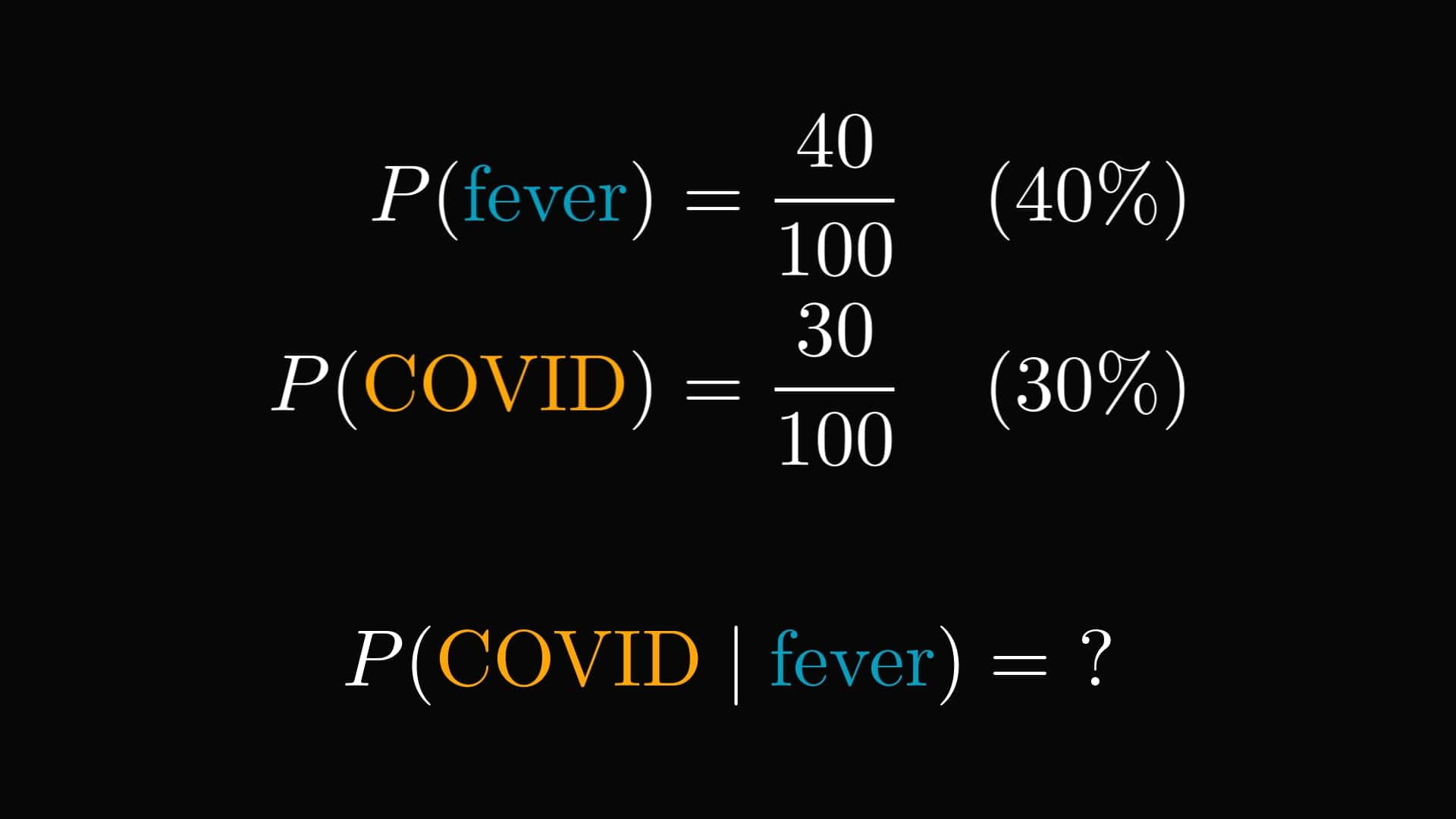By taking a more detailed look, we notice that $24$ people with a fever also got COVID.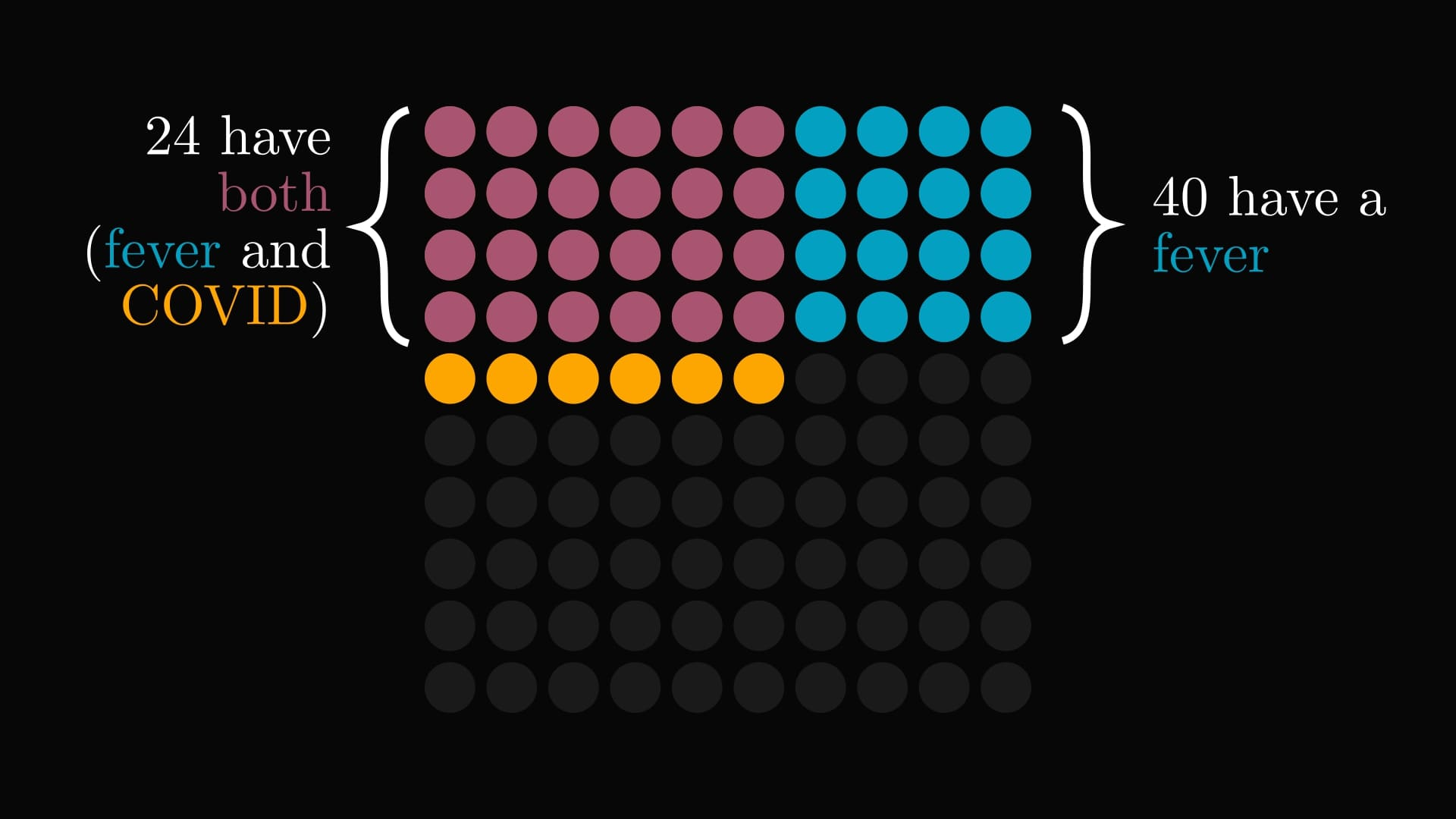Thus, we can compute the conditional probability by focusing only on people with a fever.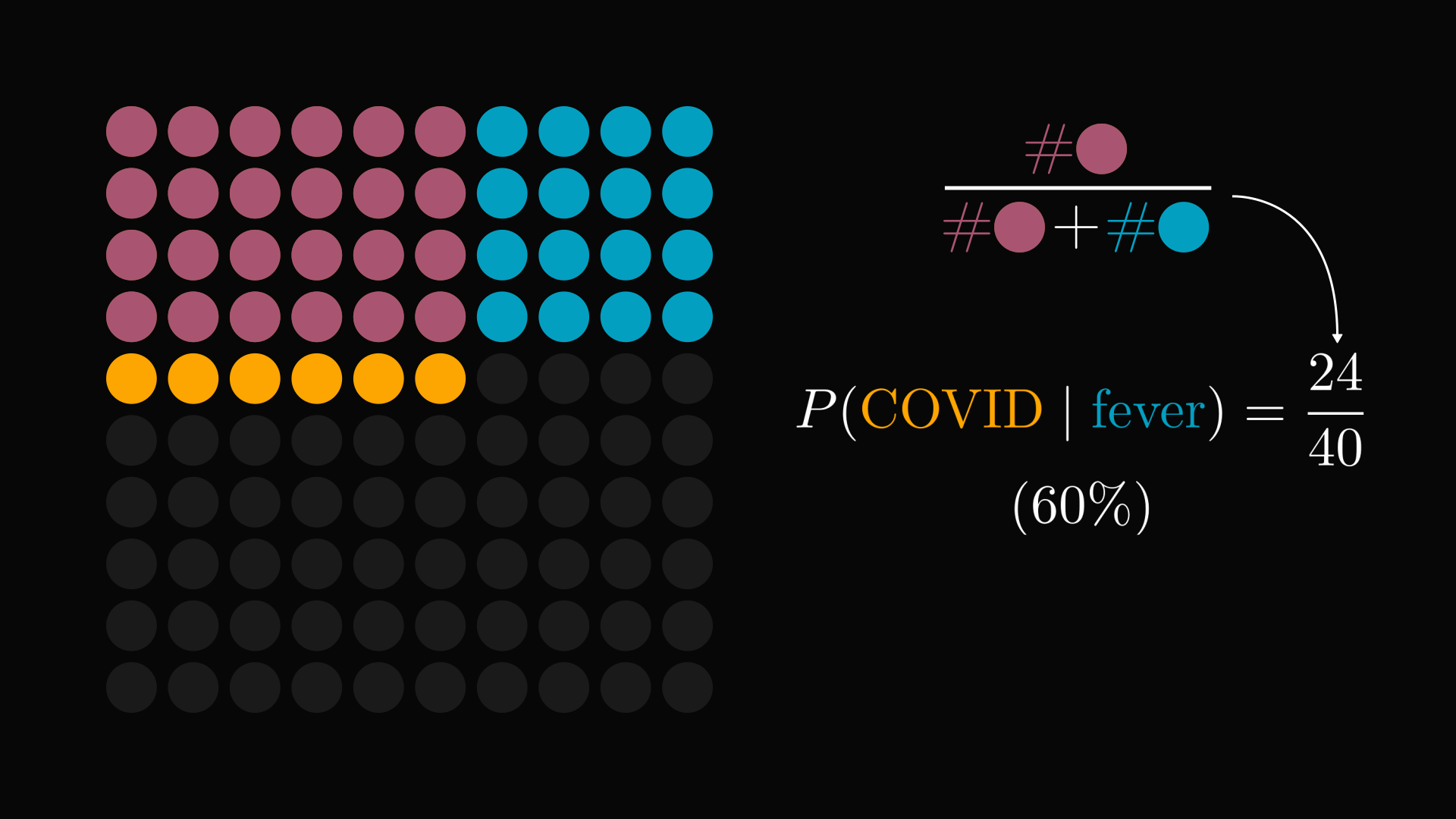Using a similar logic, we get that if the patient has no fever, the probability of COVID drops to $10\%$!

Quite a difference between our model with no prior information.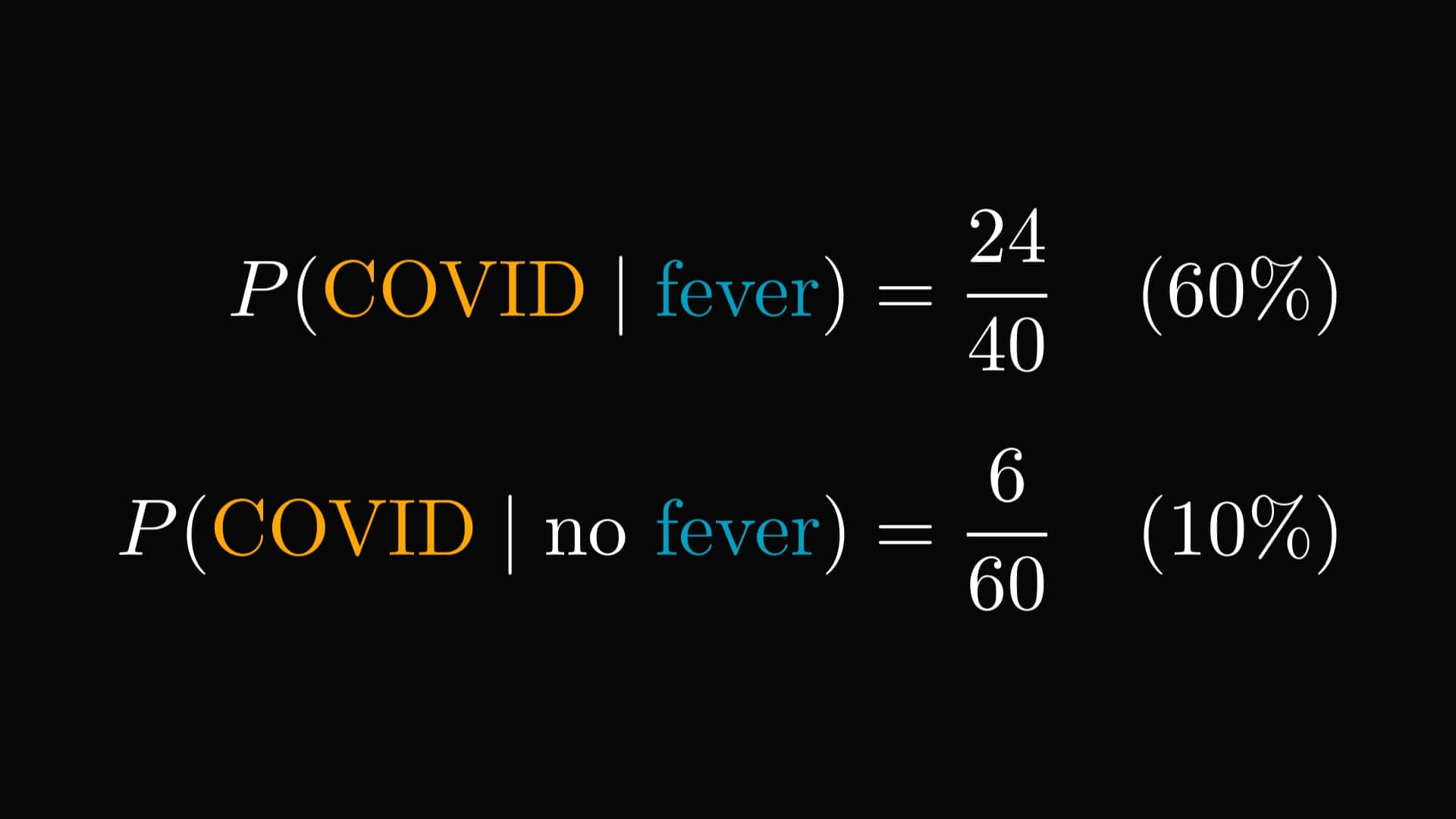Conditional probability restricts the event space, thus providing a more refined picture. This gives better models, leading to better decisions.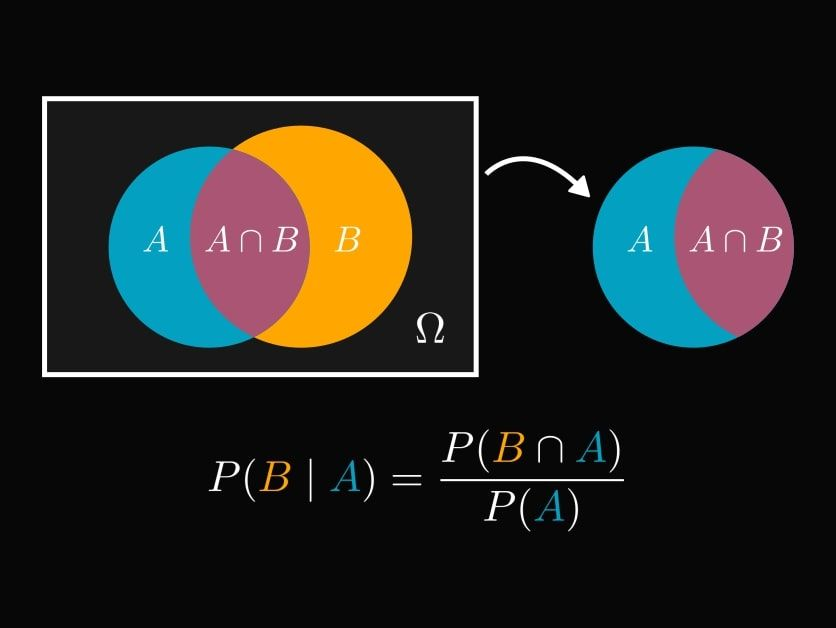(The numbers in this post are all made up. There are much more precise methods for actual COVID diagnosis.)